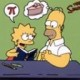## Mathematician of the Week: Thoralf Skolem

byThoralf Skolem was born on May 23, 1887 in Sandsvaer Norway. Much of his mathematical research involved the interaction of logic and algebra, including work on lattice theory and set theory. He is probably best remembered in connection with the Lowenheim-Skolem Theorem, and Skolem’s Paradox.

One version of the Lowenheim-Skolem Theorem states that any countable first order theory with an infinite model in fact has models of all infinite cardinalities.

Some examples:

• Groups: The axioms for a group constitute a first order theory. We know there are infinite models for that theory (i.e. we know that infinite groups exist which satisfy the axioms); hence by the Lowenheim-Skolem Theorem, there exist groups of all infinite cardinalities.
• Peano Arithmetic: The Peano axioms for the arithmetic of the natural numbers constitute a first order theory, with the natural numbers themselves constituting an infinite model. It follows that there are uncountable models of this theory as well; in particular, the Peano axioms fail to uniquely characterize the natural numbers, and indeed no first order theory can uniquely characterize N. More generally, no first order theory can uniquely characterize any infinite mathematical object. This fact can be used to prove that a particular property is not a first order property. For example, since the field of the real numbers R is the unique complete linearly ordered field, the notion of completeness cannot be described in terms of first order logic in the language of fields.
• Zermelo-Frankel Set Theory: The Zermelo-Frankel axioms for set theory, the putative foundation for 20th century mathematics, constitute a first order theory. If the ZF axioms are consistent (if the axioms of set theory have an infinite model), then the Lowenheim-Skolem Theorem shows that ZF has a countable model, a universe which is countable, but internally satisfies all of the properties of ZF.

The existence of a countable model of set theory is sometimes referred to as Skolem’s Paradox, for within ZF one can prove the existence of uncountable sets, and any model of ZF would contain sets that from the perspective of the model are uncountable.

How could such a thing occur?

Countability is the property of being able to be placed in one to one correspondence with N. For an “uncountable” set U within a countable model of set theory, we can see externally that the set U must be countable (there exists a bijection between U and N), but the bijection itself is not an object in the model. Hence in the model’s interpretation of set theory, U is uncountable.

Skolem remained active in research up until his death in 1963 at the age of 75.

Other mathematicians with significant anniversaries for the week of May 18 through May 24:

May 18: Birthdays of Oliver Heaviside (1850) and Bertrand Russell (1872)

May 19: Birthday of Serge Lang (1927); death anniversary of Francis Maseres (1824)

May 20: Birthday (1861) and death anniversary (1943) of Henry White

May 21: Birthdays of Albrecht Dürer (1471), Gaspard-Gustave de Coriolis (1792), and Edouard Goursat (1858); death of Ernst Zermelo (1953)

May 22: Birthday of Albrecht Fröhlich (1916)

May 23: Birthday of Thoralf Skolem (1887); death of Augustin-Louis Cauchy (1857)

May 24: Birthday of William Chauvenet (1820); deaths of Nicolaus Copernicus (1543) and Sylvestre Lacroix (1843)

Source for dates and biographical info on Skolem: The MacTutor History of Mathematics Archive.

### 3 Responses to “Mathematician of the Week: Thoralf Skolem”

1.Ξ Says:

Can I just say that, even though I like paradoxes [even ones I have to go back to analysis to be able to think about], I’m disappointed that Thoralf Skolem doesn’t have a pop-song a la Mascheroni/My Sharona.

2.PlusC Says:

Nikky Copernicus died on my birthday… that puts a damper on it =(

3.Mathematician of the week: Jules Lissajous « 360 Says:

[…] 26: Birthday of Leopold Löwenheim  (Löwenheim-Skolem Theorem); death of George Udny Yule  […]